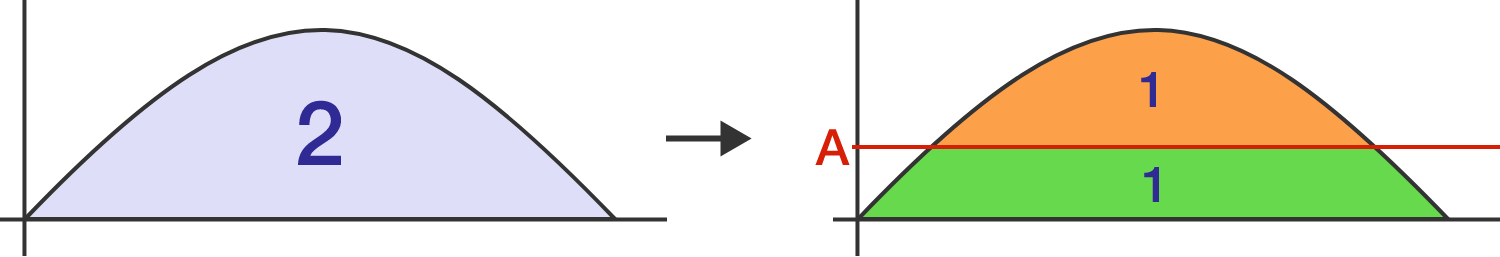# Half sine

Calculus Level 4

Notably, the area under the curve $y=\sin(x)$ over a semiperiod is exactly 2 since $\int_0^\pi\sin(x)\,dx=-\cos(x)\Big|_0^\pi=2.$ Naturaly then, there is a point $(0,A)$ on the $y$-axis such that a horizontal line through it divides the above area into two regions of area 1, as shown.

What is $A?$×